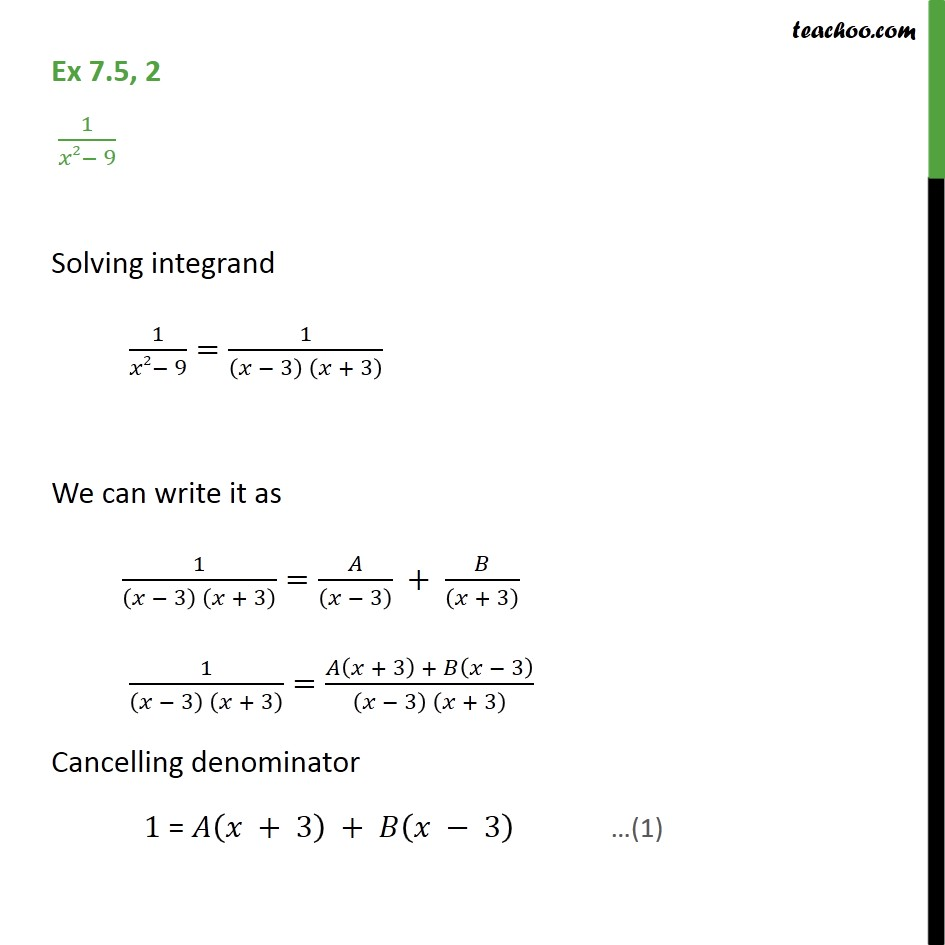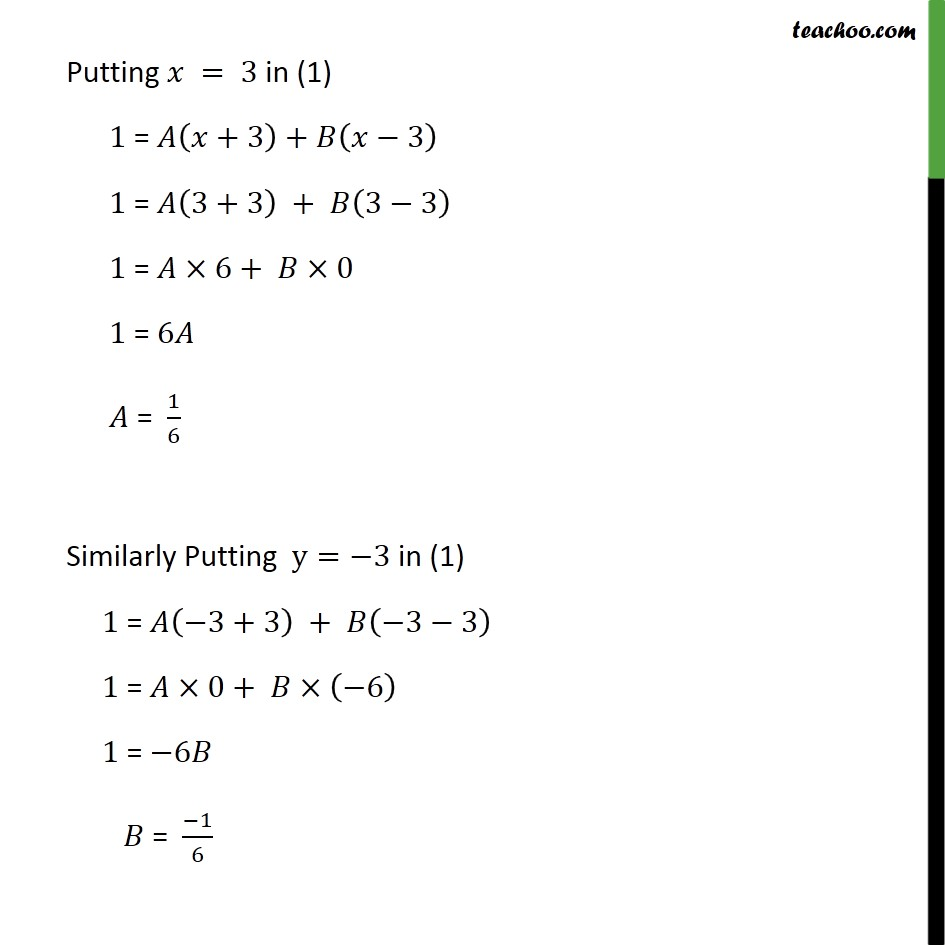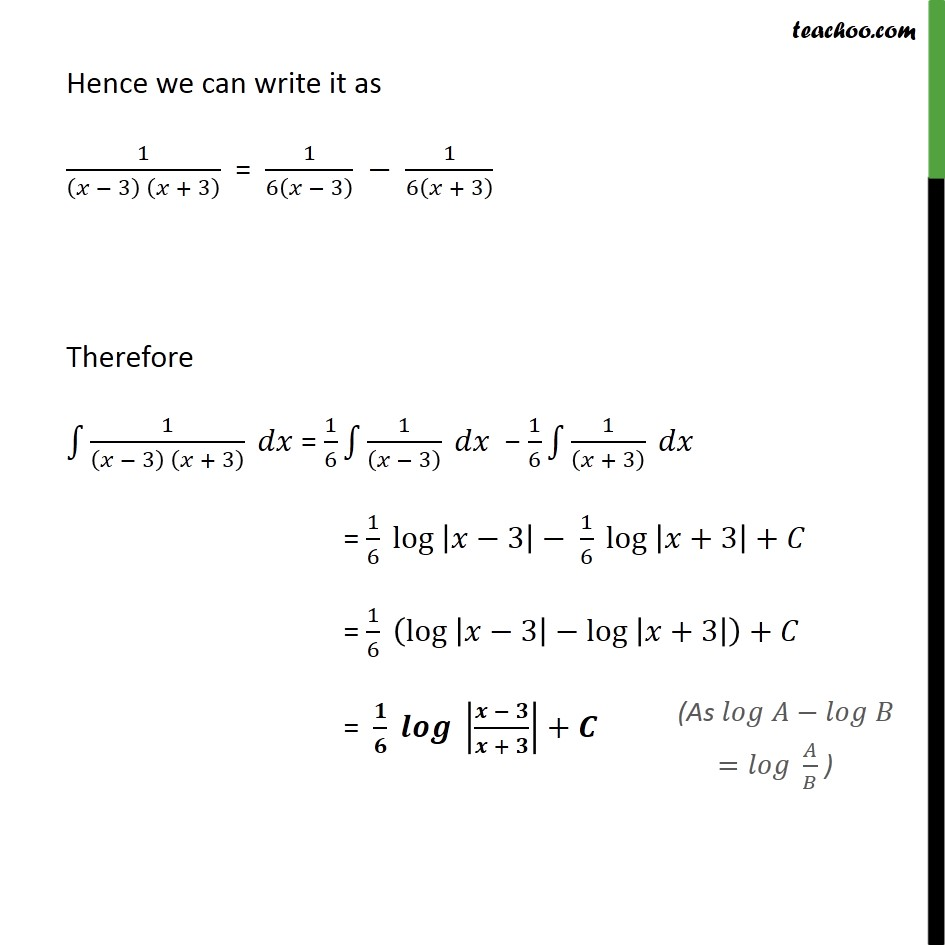Ex 7.5

Chapter 7 Class 12 Integrals
Serial order wiseLearn in your speed, with individual attention - Teachoo Maths 1-on-1 Class

### Transcript

Ex 7.5, 2 1/(𝑥2− 9) Solving integrand 1/(𝑥2− 9)=1/((𝑥 − 3) (𝑥 + 3) ) We can write it as 1/((𝑥 − 3) (𝑥 + 3) )=𝐴/((𝑥 − 3) ) + 𝐵/((𝑥 + 3) ) 1/((𝑥 − 3) (𝑥 + 3) )=(𝐴(𝑥 + 3) + 𝐵(𝑥 − 3))/((𝑥 − 3) (𝑥 + 3) ) " " Cancelling denominator 1 = 𝐴(𝑥 + 3) + 𝐵(𝑥 − 3) Putting 𝑥 = 3 in (1) 1 = 𝐴(𝑥+3)+𝐵(𝑥−3) 1 = 𝐴(3+3) + 𝐵(3−3) 1 = 𝐴×6+ 𝐵×0 1 = 6𝐴 𝐴 = 1/6 Similarly Putting y=−3 in (1) 1 = 𝐴(−3+3) + 𝐵(−3−3) 1 = 𝐴×0+ 𝐵×(−6) 1 = −6𝐵 𝐵 = (−1)/6 Hence we can write it as 1/((𝑥 − 3) (𝑥 + 3) ) = 1/6(𝑥 − 3) − 1/6(𝑥 + 3) Therefore ∫1▒1/((𝑥 − 3) (𝑥 + 3) ) 𝑑𝑥 = 1/6 ∫1▒1/((𝑥 − 3) ) 𝑑𝑥 − 1/6 ∫1▒1/((𝑥 + 3) ) 𝑑𝑥 = 1/6 〖log 〗⁡|𝑥−3|− 1/6 〖log 〗⁡|𝑥+3|+𝐶 = 1/6 (〖log 〗⁡|𝑥−3|−〖log 〗⁡|𝑥+3| )+𝐶 = 𝟏/𝟔 〖𝒍𝒐𝒈 〗⁡|(𝒙 − 𝟑)/(𝒙 + 𝟑)|+𝑪